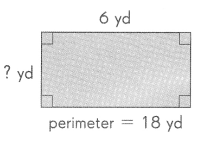# Math in Focus Grade 4 Chapter 12 Practice 2 Answer Key Rectangles and Squares

This handy Math in Focus Grade 4 Workbook Answer Key Chapter 12 Practice 2 Rectangles and Squares detailed solutions for the textbook questions.

## Math in Focus Grade 4 Chapter 12 Practice 2 Answer Key Rectangles and Squares

Find the perimeter of each figure.

Example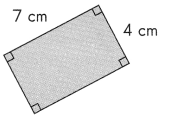Perimeter of rectangle
= 7 + 4 + 7 + 4
= 22 cm
The perimeter of the rectangle is 22 centimeters.

Question 1.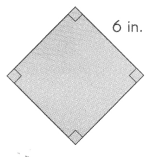Perimeter of square = 4 × ___________
= _________ in.
The perimeter of the square is ___________inches.
In square all four sides are equal.
Perimeter of square = 4 × 6 in
= 24 in.
The perimeter of the square is 24 inches.

Example

The perimeter of a square flower garden is 20 feet. Find the length of one side of the flower garden.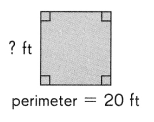Length of one side = perimeter ÷ 4
= 20 ÷ 4
= 5 ft
The length of one side of the flower garden so 5 feet.

Question 2.
The perimeter of a square building is 160 yards. Find the length of one side of the building.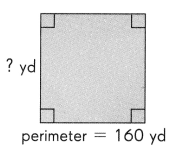Perimeter = 160 yards
Length of one side = perimeter ÷ 4
= 160 yards ÷ 4
= 40 yards
The length of one side of the building is 40 yards.

Question 3.
A square field has a perimeter of 44 meters. Find the length of one side of the field.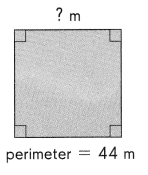Perimeter = 44 m
Length of one side = perimeter ÷ 4
= 44 m ÷ 4
= 11 m
The length of one side of the field is 11 m.

Question 4.
The perimeter of a rectangular town is 32 miles. Its width is 5 miles. Find the length.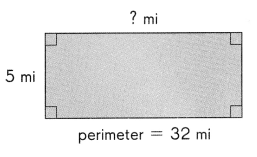From the above image we can have a data of width and perimeter.
Length = ?
width = 5 mi
perimeter = 32 mi
Perimeter of the rectangular town = length + width + length + width
32 mi = l + 5 mi + l + 5 mi
32 mi = 2l + 10 mi
32 mi – 10 mi = 2l
22 mi = 2l
11 mi = l
The length of the rectangular town is 11 miles.

Question 5.
The perimeter of a rectangle is 24 centimeters. Its length is 9 centimeters. Find the width.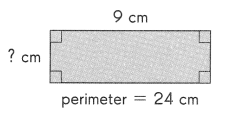From the above image we can have a data of length and perimeter.
Length = 9 cm
width = ?
perimeter = 24 cm
Perimeter of the rectangle = length + width + length + width
24 cm = 9 cm + w + 9 cm + w
24 cm= 18 cm + 2w
24 cm – 18 cm = 2w
6 cm = 2w
3 cm = w
The width of the rectangle is 3 cm.

Question 6.
The perimeter of a rectangular garden is 18 yards. Its length is 6 yards. Find the width.